Download the eSaral app and start learning from Kota's top IITians and doctors.

# NCERT Solutions for Class 9 Maths chapter 8 - Quadrilaterals - eSaral

Class 9NCERT Solutions for Class 9 Maths chapter 8 - Quadrilaterals-eSaral provides,solutions for a crucial topic in the Maths syllabus. This chapter deals with the study of quadrilaterals, their properties, angles, sides, diagonals, and theorems related to them. It is important for students to have a clear understanding of this topic as it lays the foundation for further studies in geometry.

The NCERT Solutions provided by eSaral for this chapter are designed to help students in their exam preparation by providing comprehensive coverage of all the important topics. The solutions are presented in an easy-to-understand manner and include a large number of practice questions with step-by-step solutions.

By using eSaral's NCERT Solutions for Class 9 Maths chapter 8 - Quadrilaterals, students can improve their problem-solving skills, boost their confidence, and achieve better grades in exams.

In this article, we have listed NCERT solutions for class 9 Maths Chapter 8 in PDF that is prepared by Kota’s top IITian’s Faculties by keeping Simplicity in mind.

If you want to learn and understand class 9 Maths chapter 8 "Quadrilaterals" in an easy way then you can use these solutions PDF.

If you want to score high in your class 9 Maths Exam then it is very important for you to have a good knowledge of all the important topics, so to learn and practice those topics you can use eSaral NCERT Solutions.

So, without wasting more time Let’s start.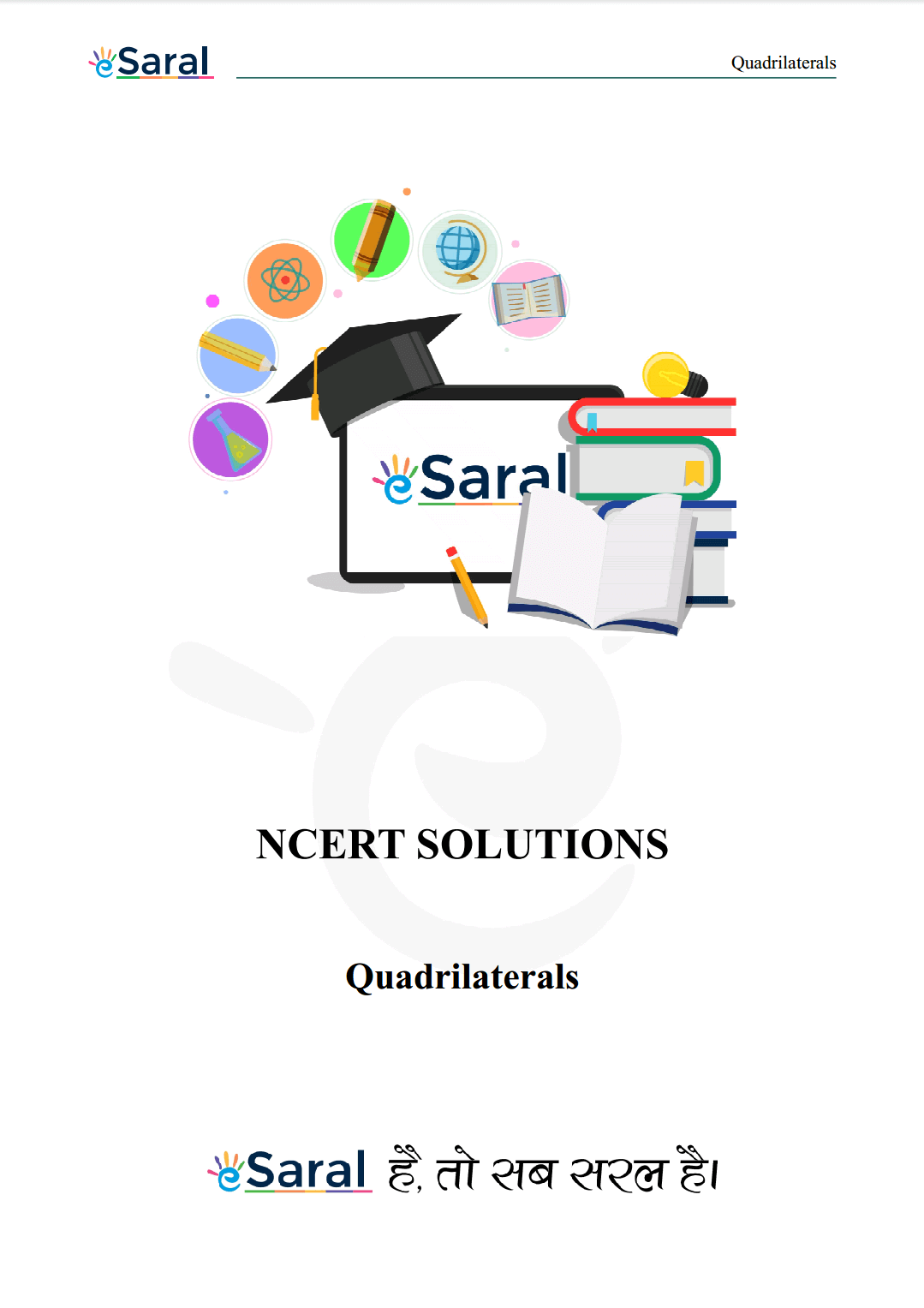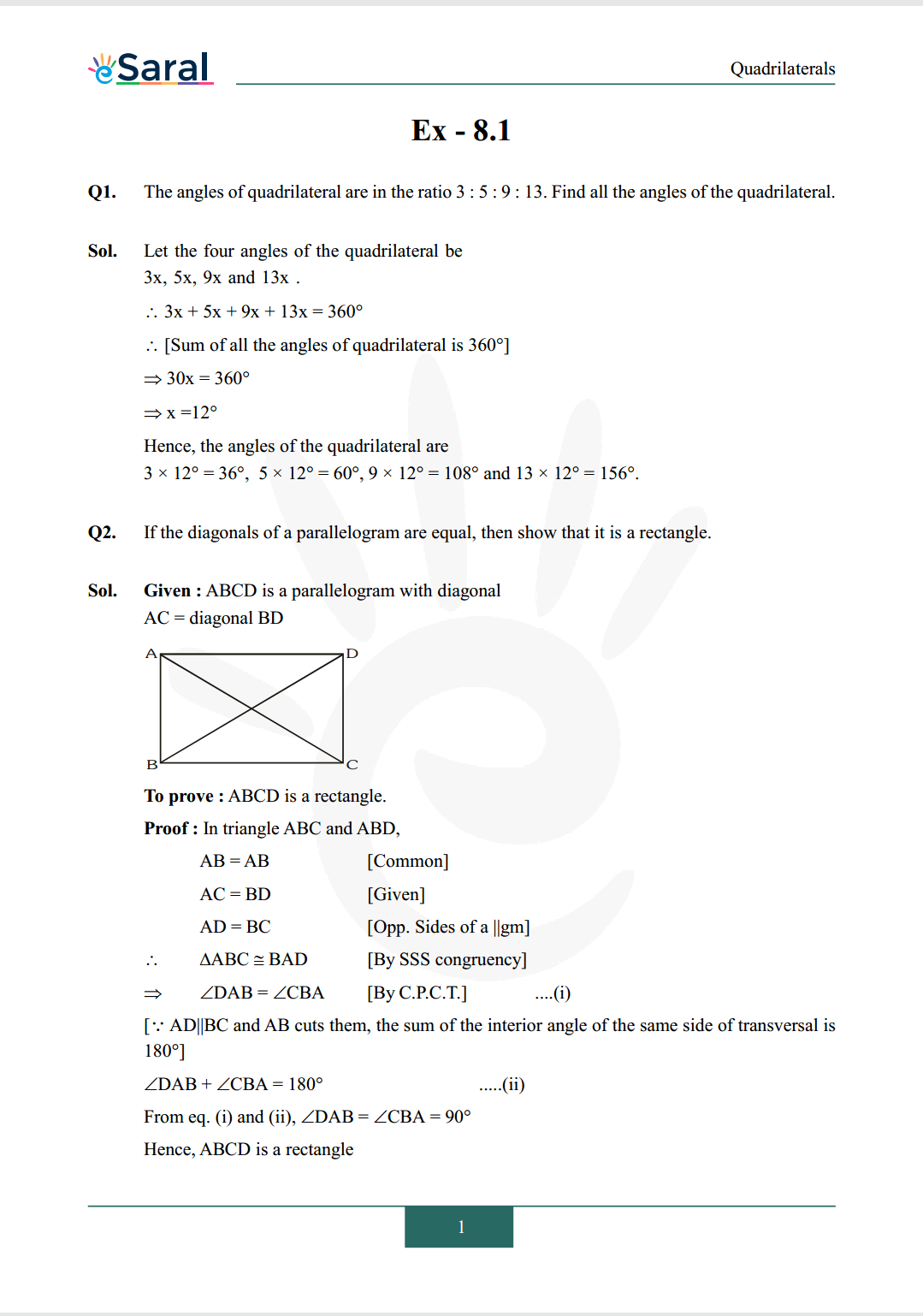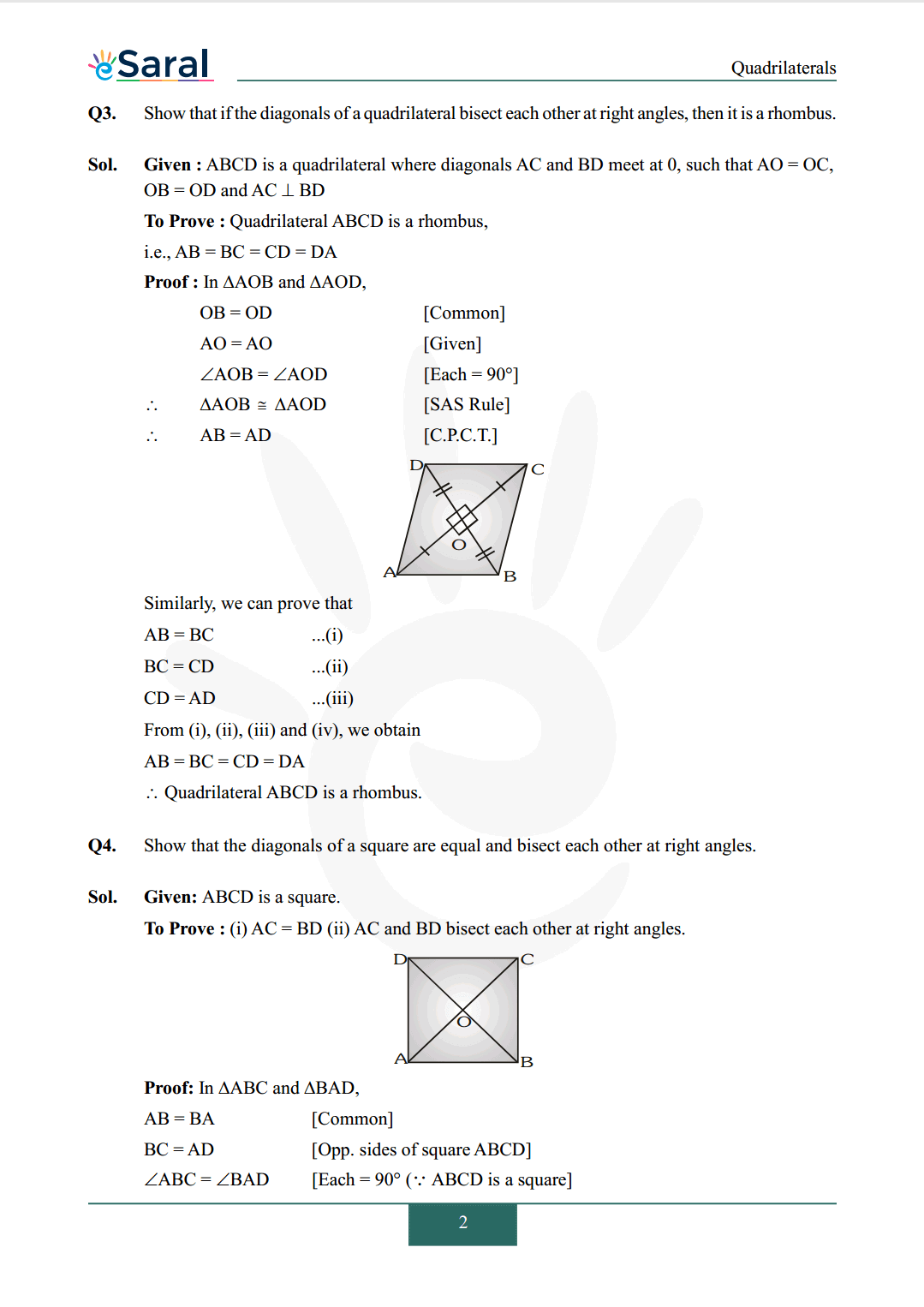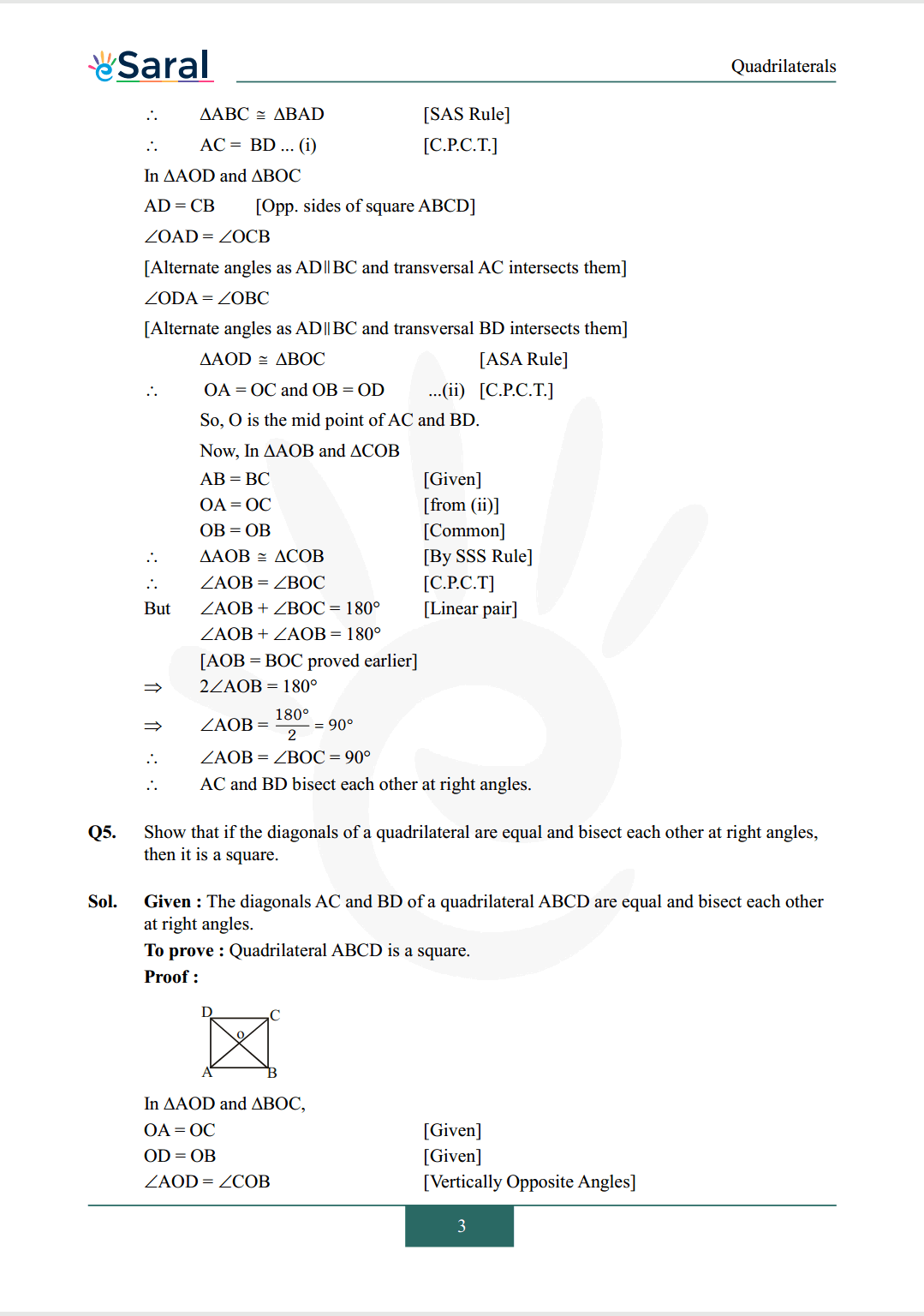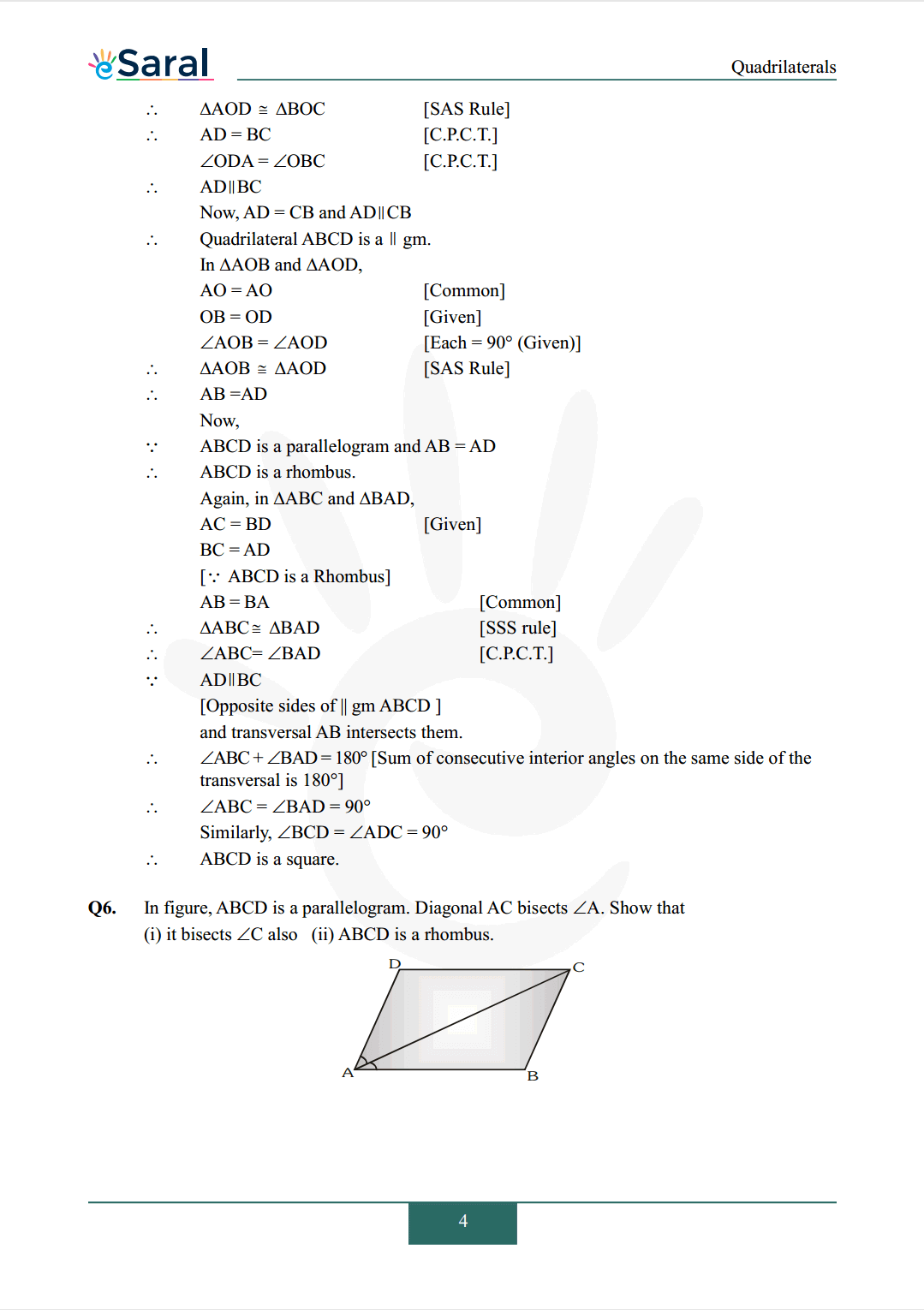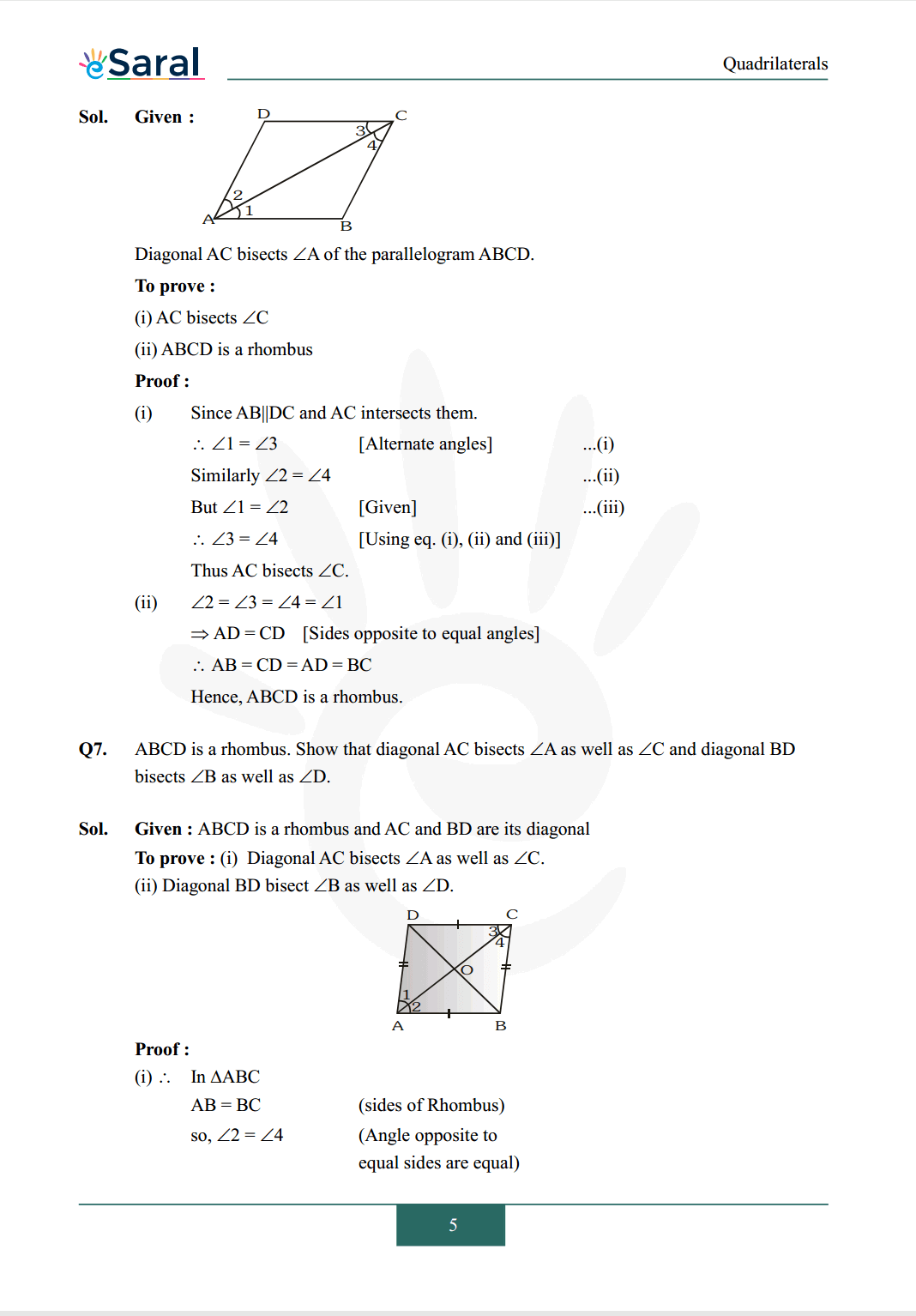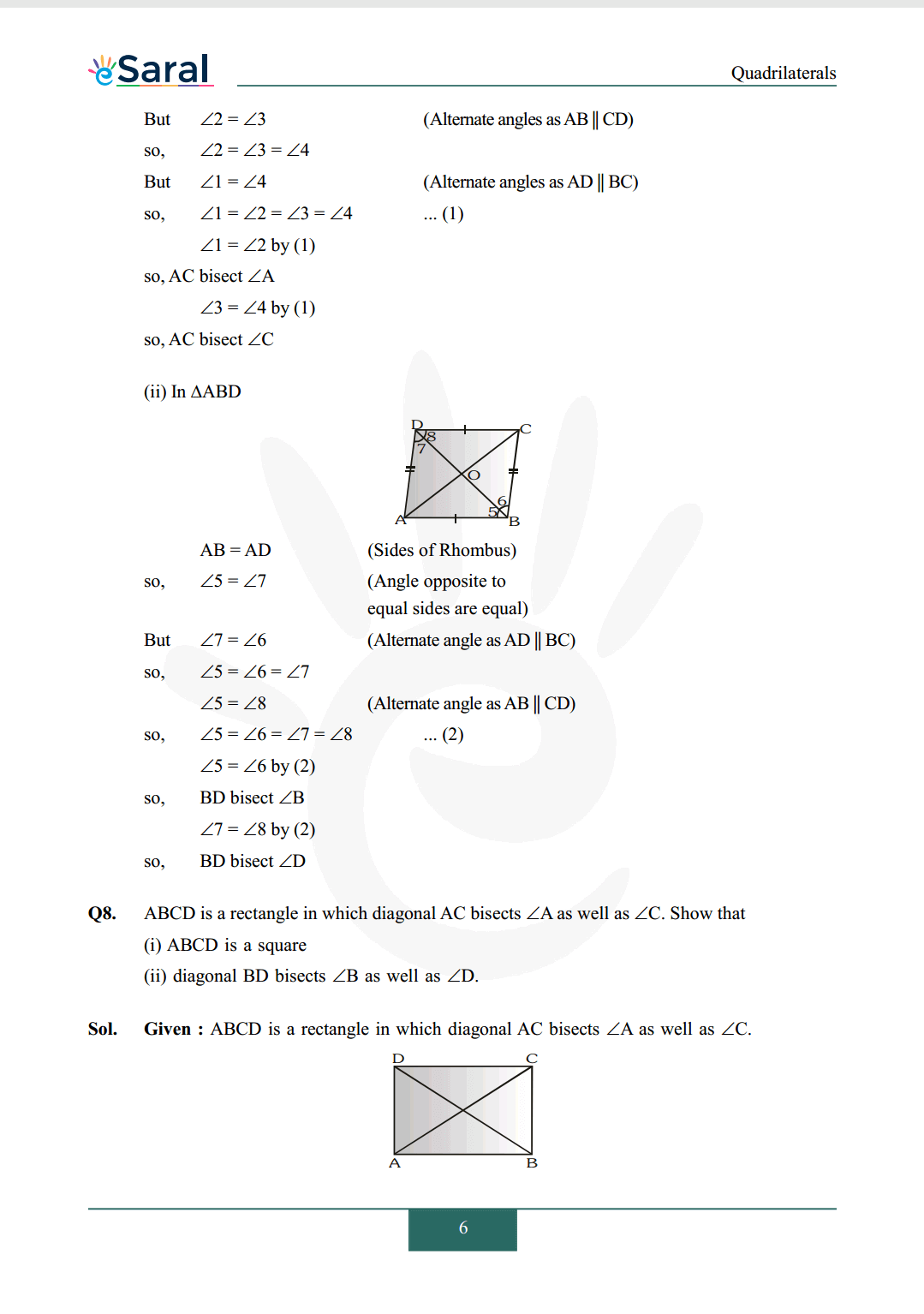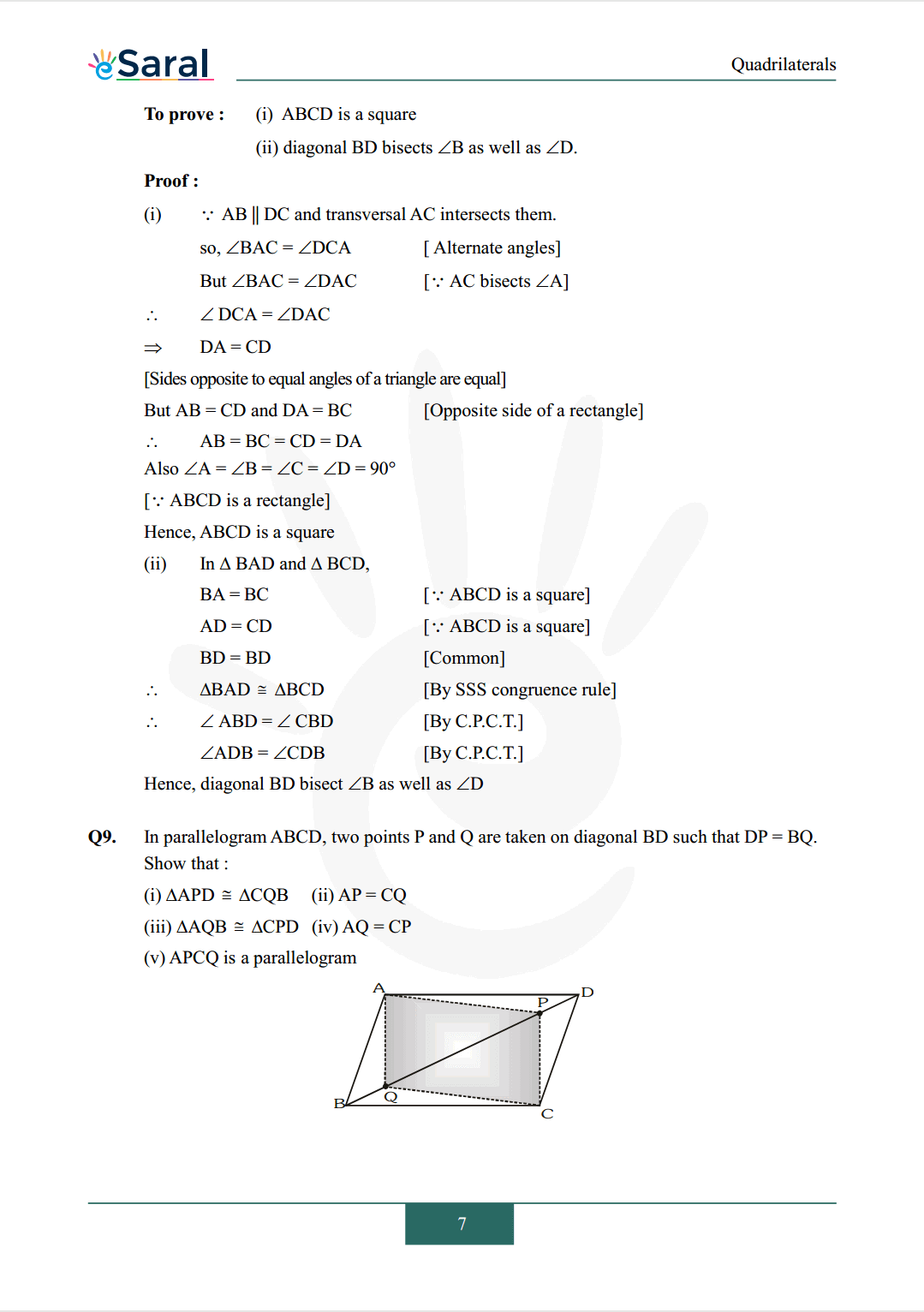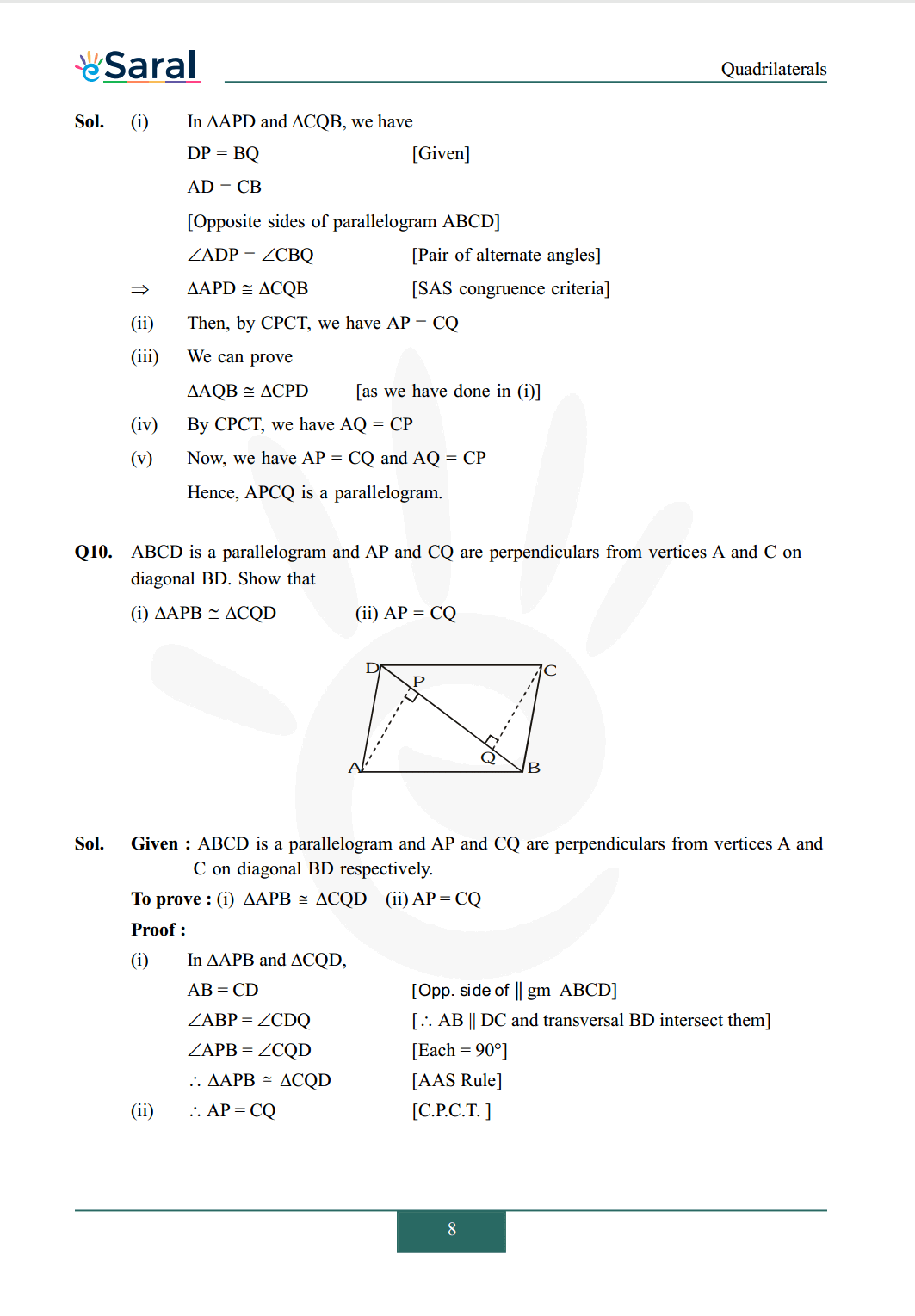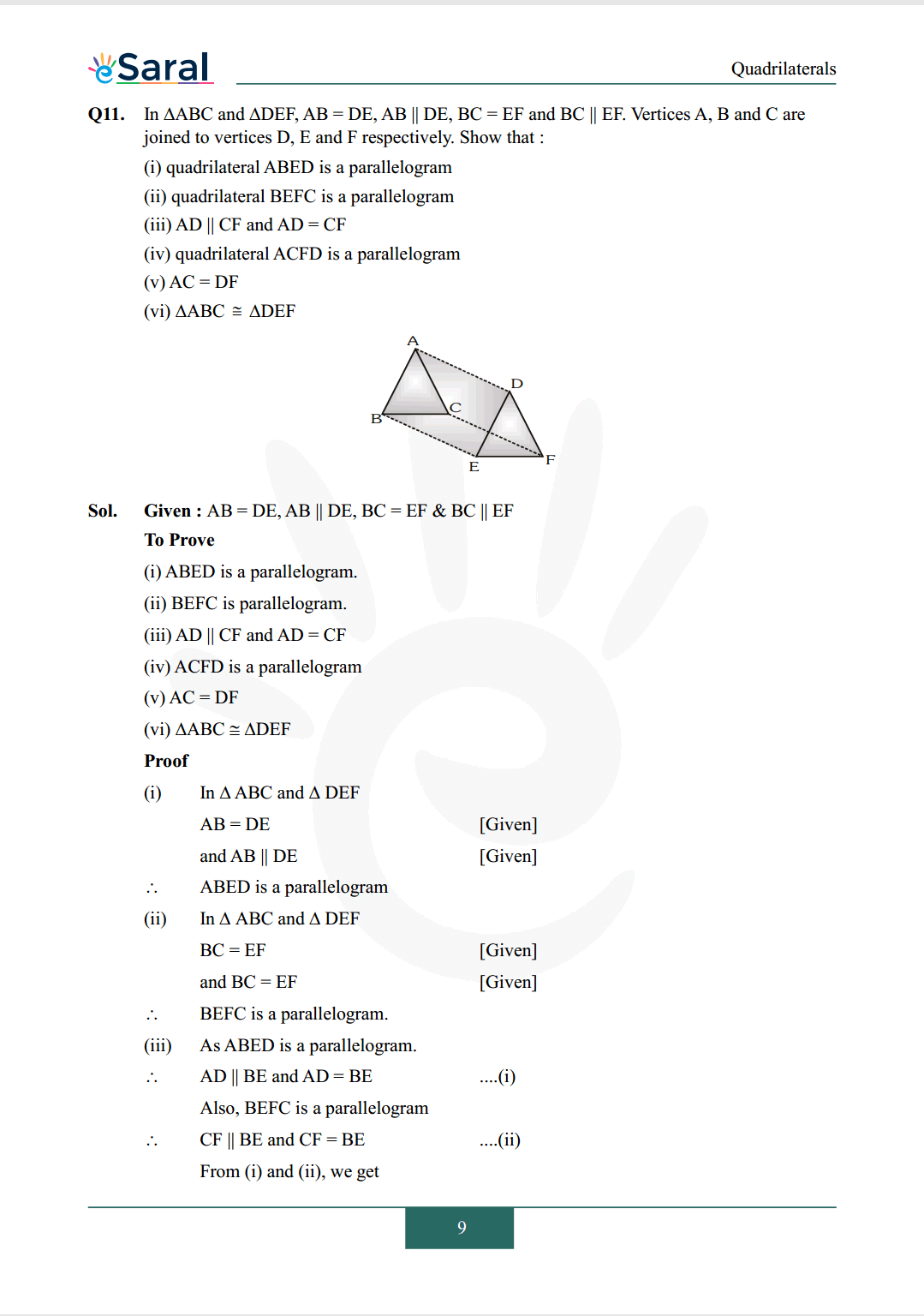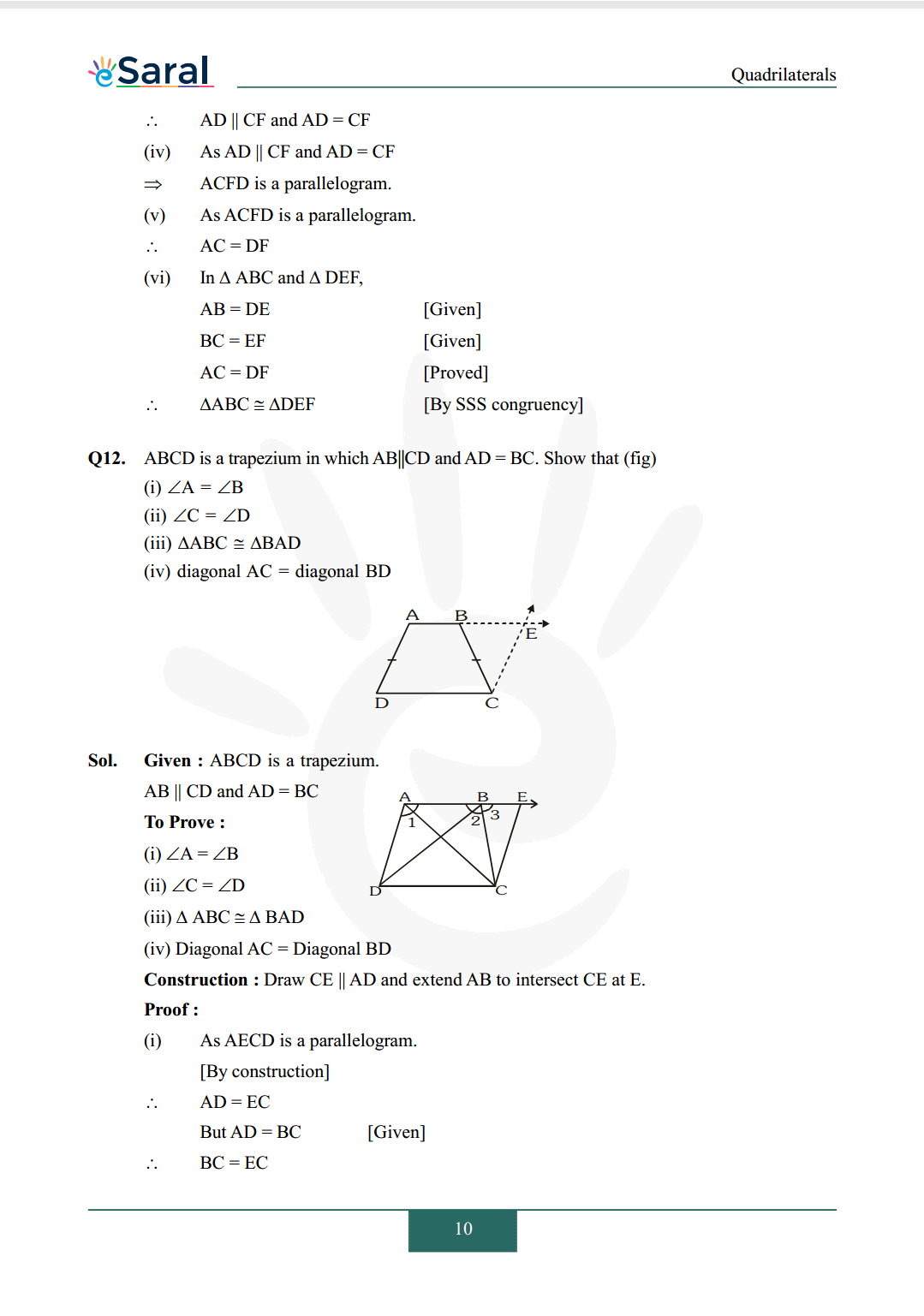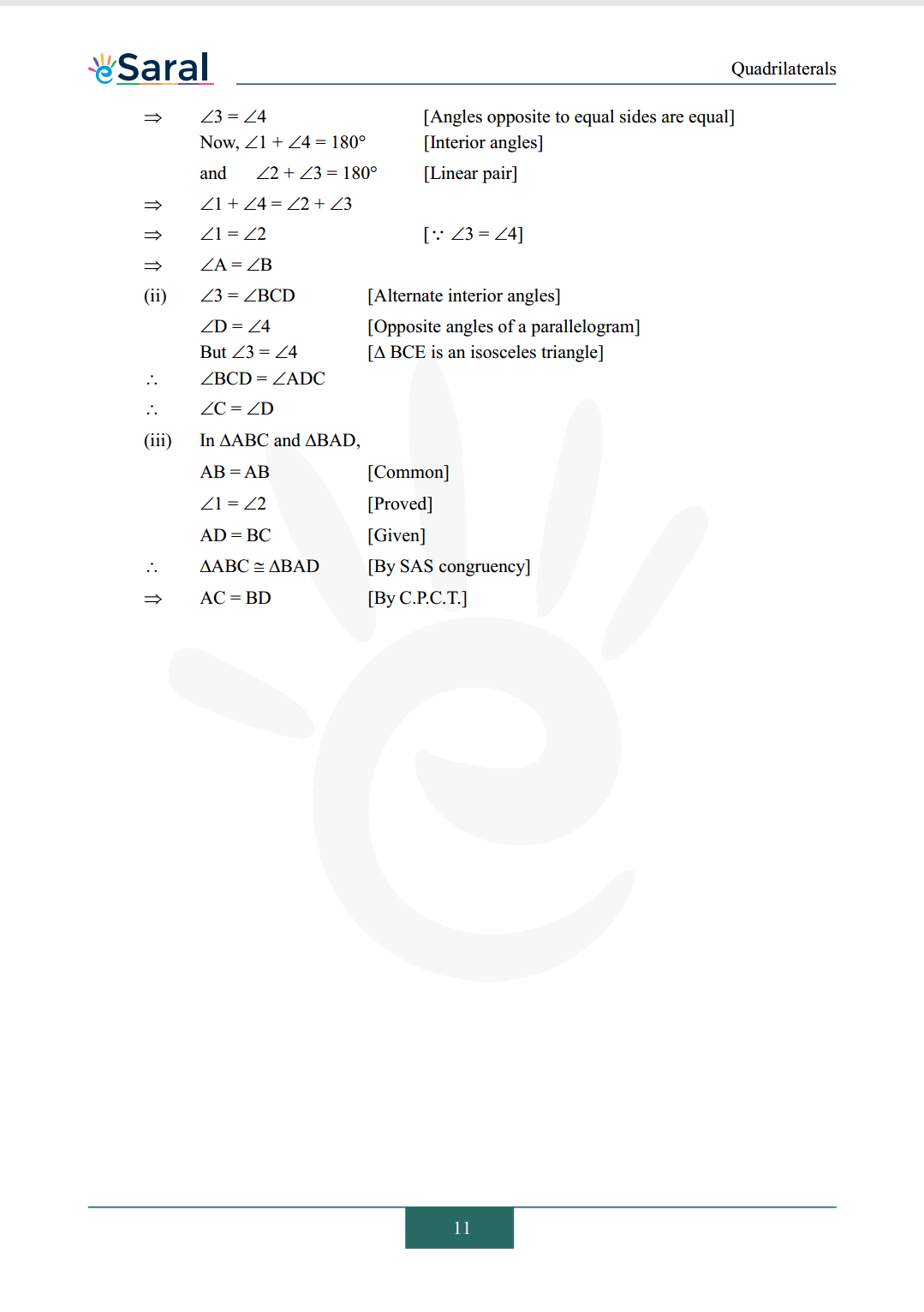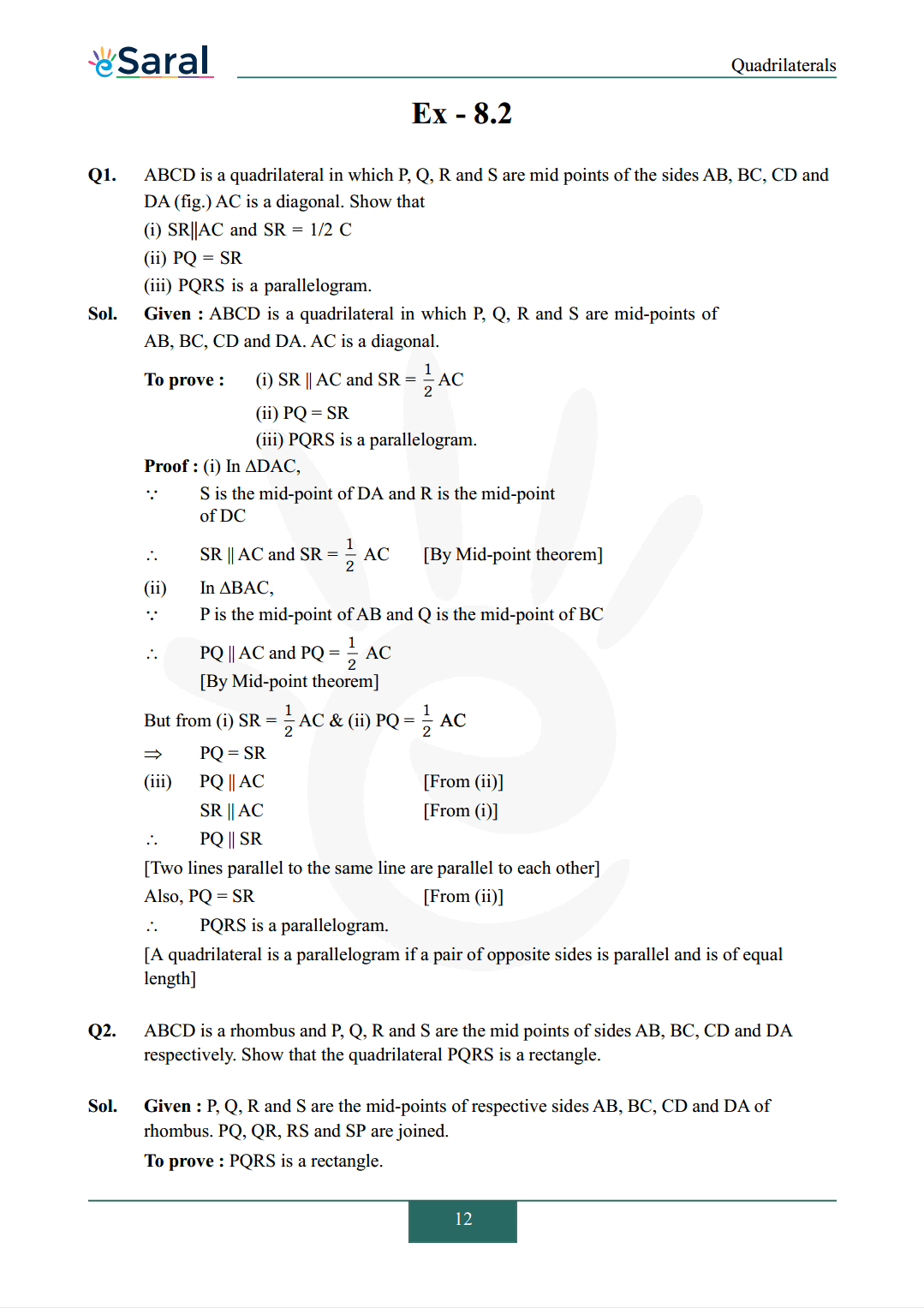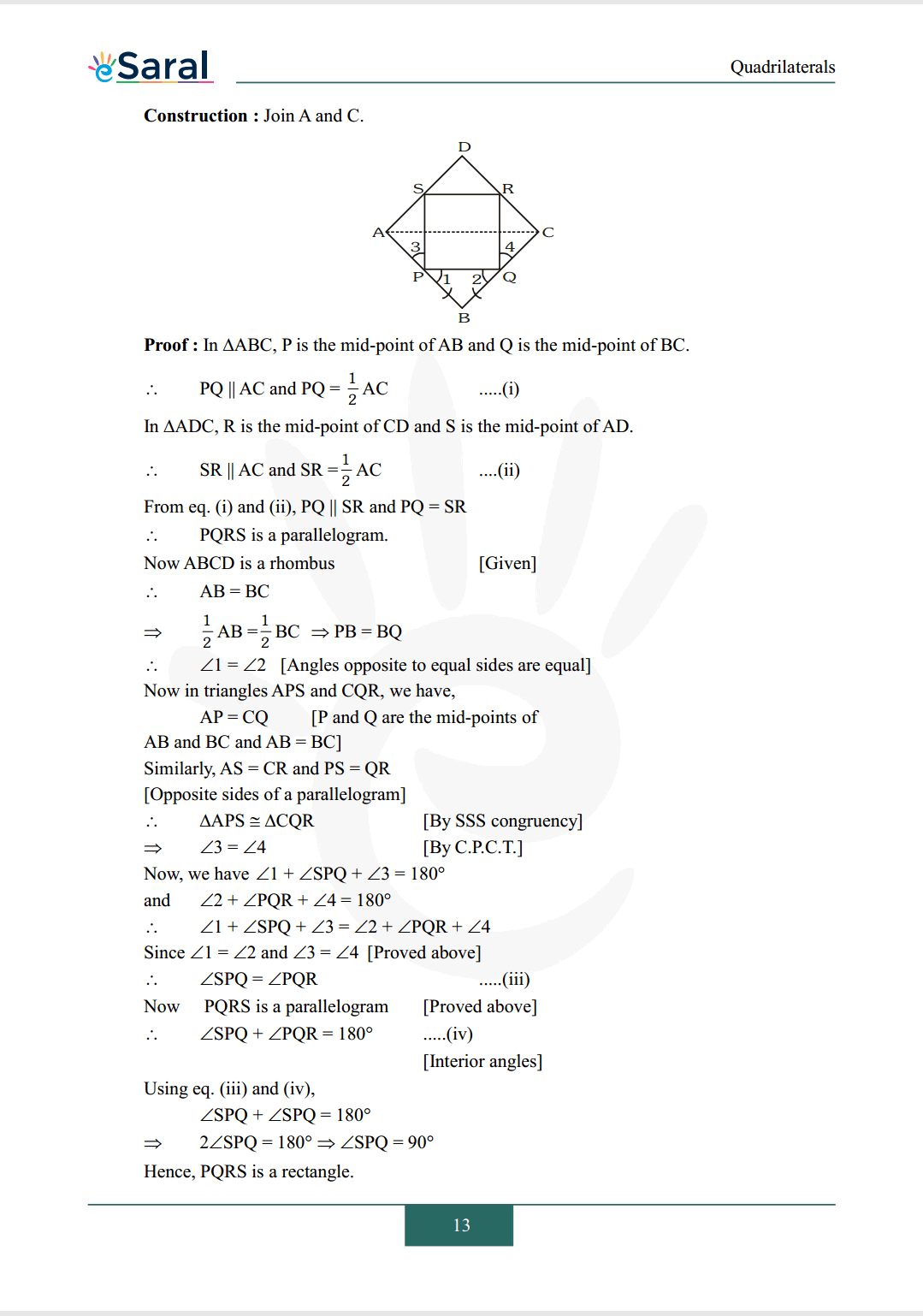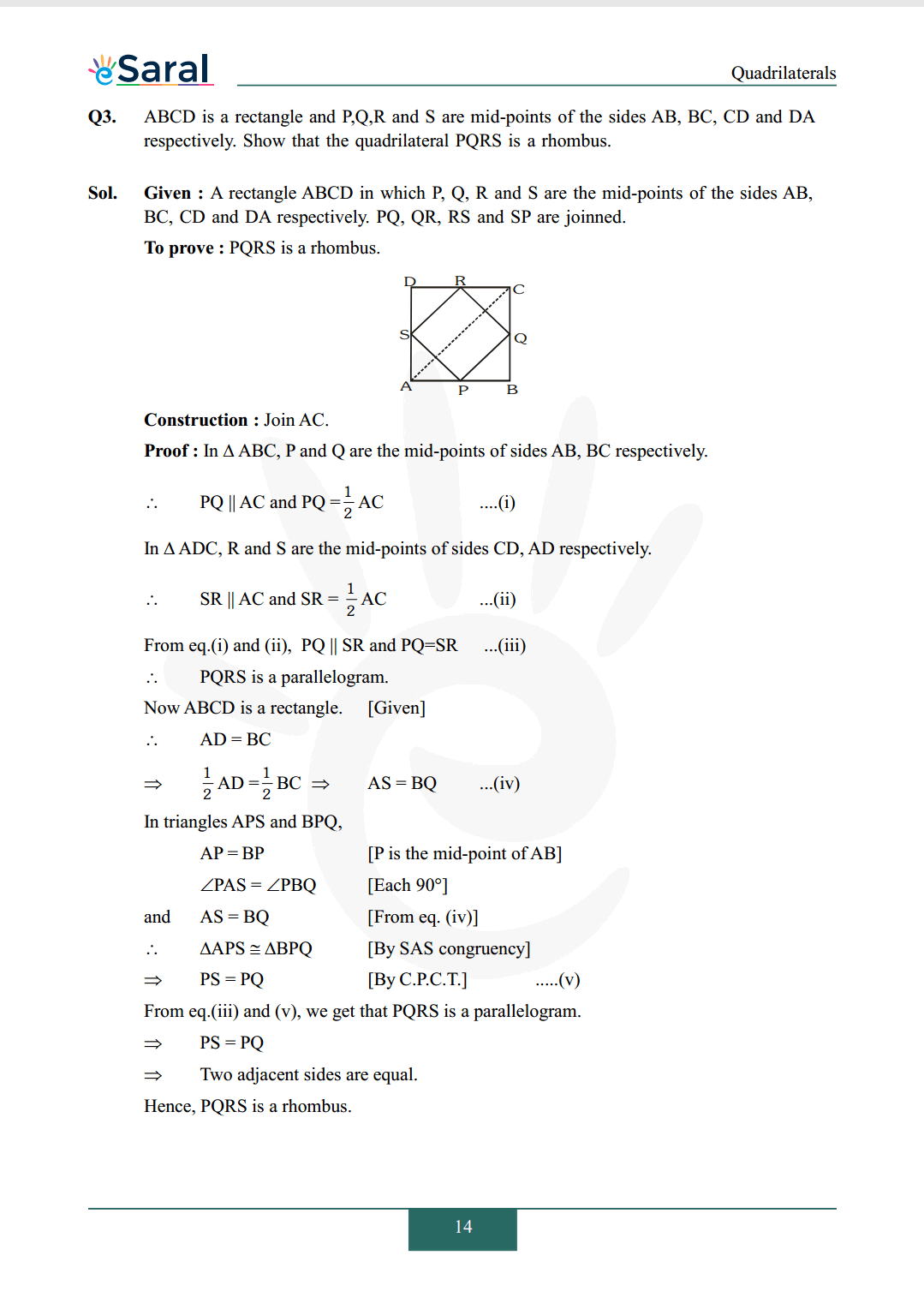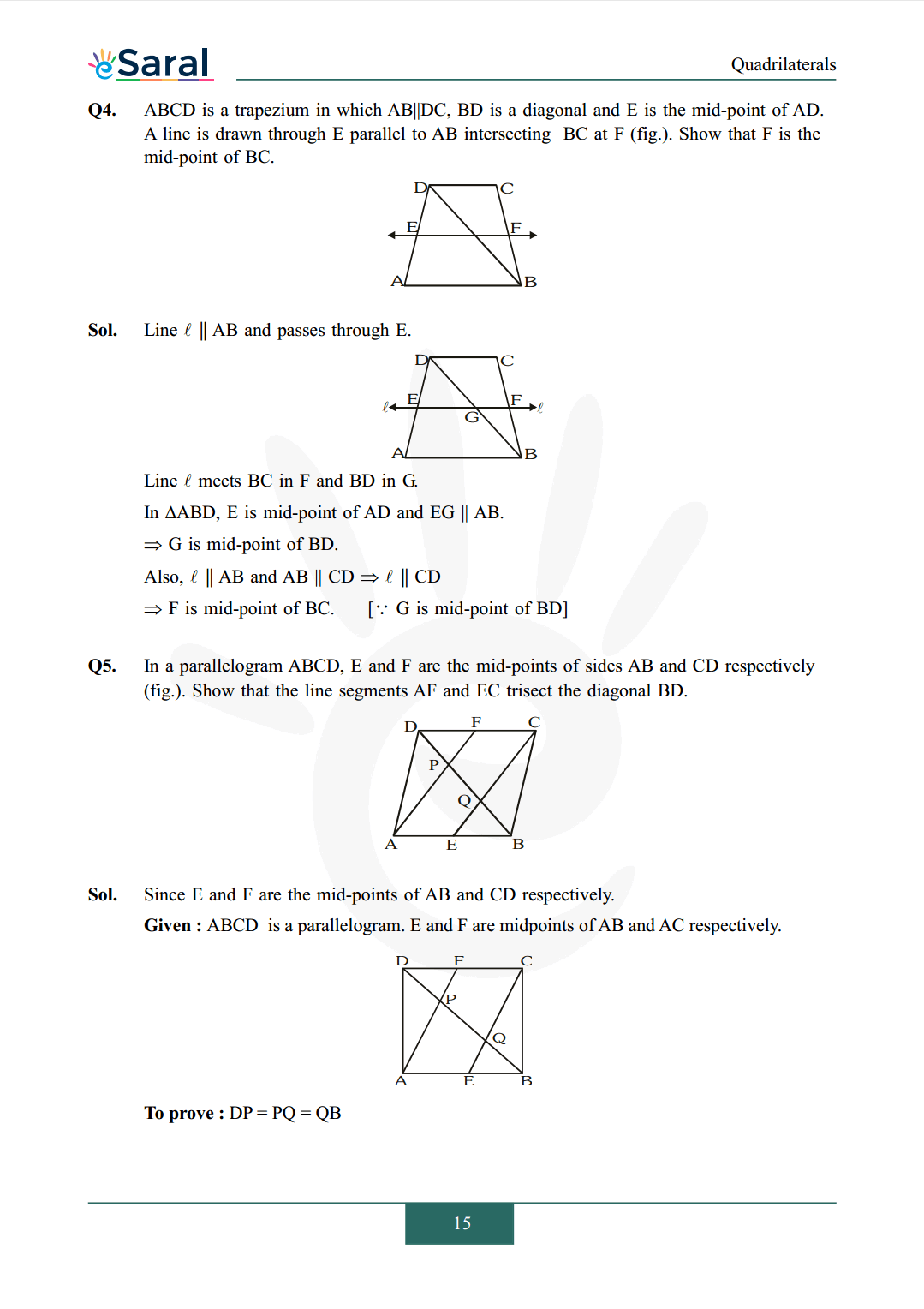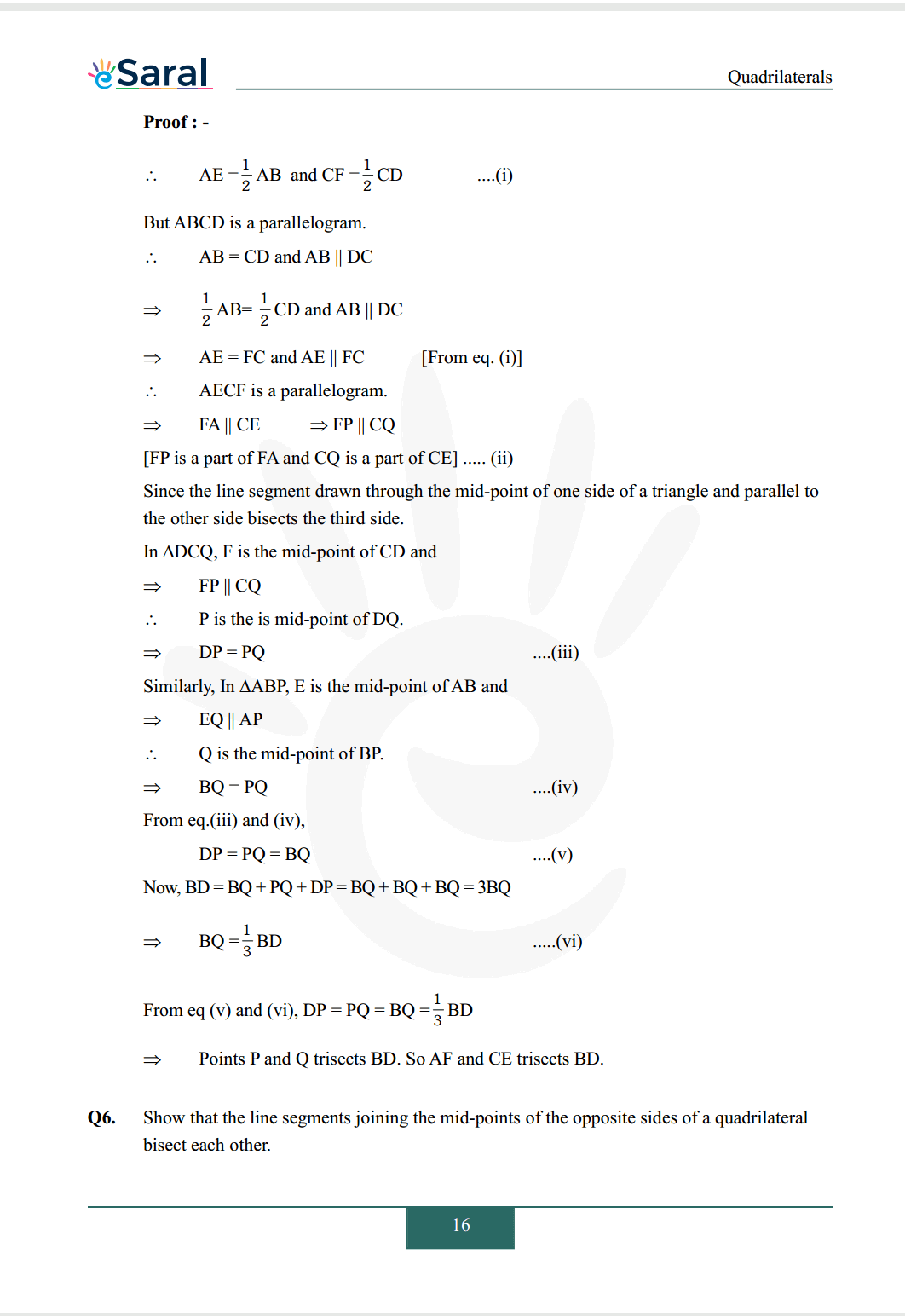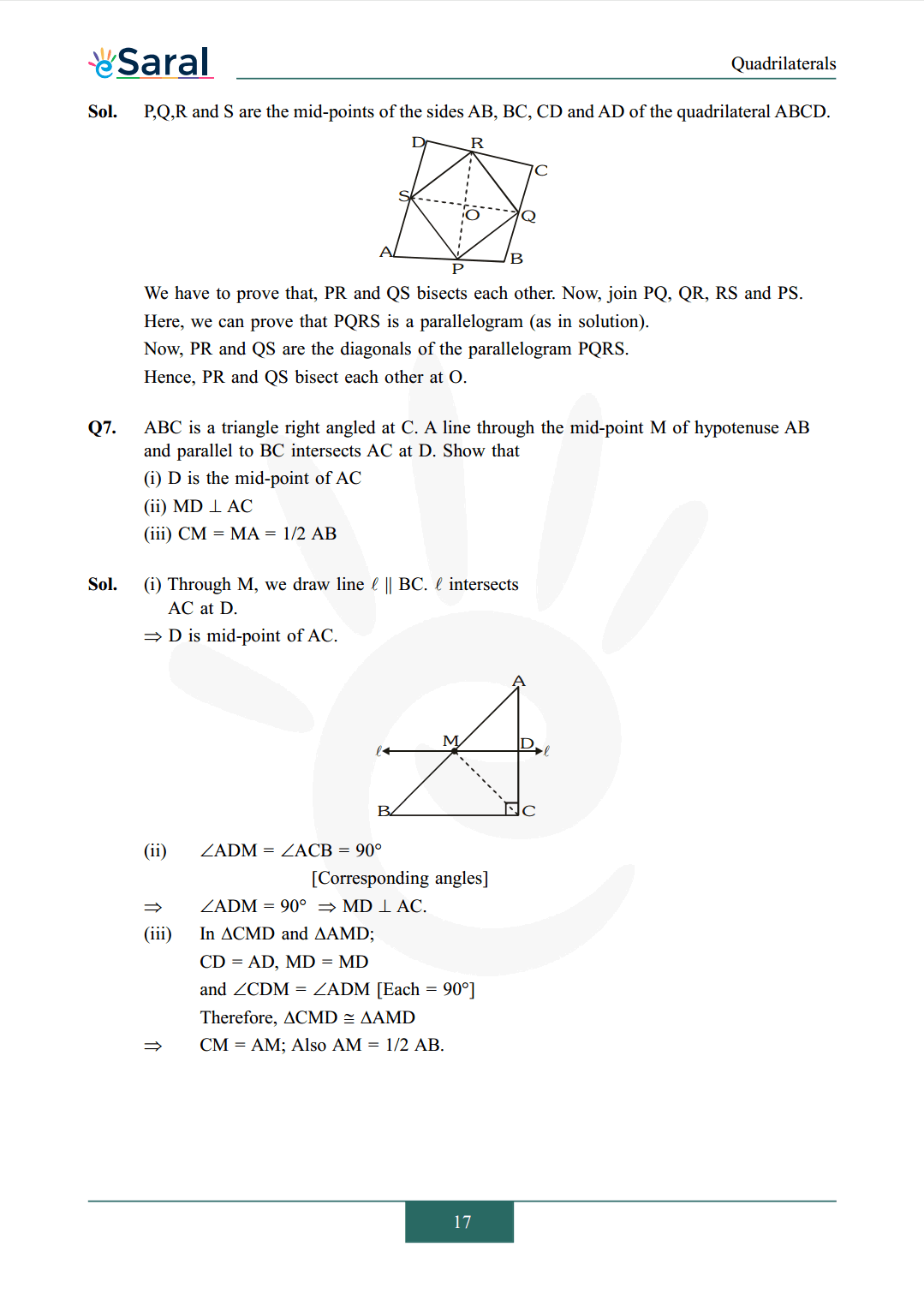Quadrilaterals are defined as polygons with four sides. They can be categorized based on their properties, such as parallel sides, congruent sides, congruent angles, and right angles. The properties of a quadrilateral are determined by its angles and sides.

### Benefits of NCERT Solutions for Class 9 Maths chapter 8 - Quadrilaterals - eSaral for students?

1. Comprehensive coverage: The NCERT solutions for Class 9 Maths chapter 8 provide a comprehensive coverage of all the important topics related to quadrilaterals. It includes various types of quadrilaterals, their properties, angles, sides, diagonals, and theorems related to them.

2. Simplified explanation: The solutions provide a simplified explanation of the concepts and theorems related to quadrilaterals. This helps students to understand the topics easily and also helps in retaining the concepts for a longer time.

3. Practice questions: The NCERT solutions include a large number of practice questions with step-by-step solutions. These practice questions are designed to test the understanding of the concepts and theorems learned by the students. They also help in improving problem-solving skills and boost confidence.

4. Time-saving: The solutions are designed in a manner that saves the students' time. They provide quick solutions to the problems, which helps in completing the homework and assignments quickly.

5. Helps in exam preparation: The NCERT solutions for Class 9 Maths chapter 8 are very helpful in exam preparation. They provide a clear understanding of the topics and also provide an idea about the types of questions that can be asked in the exam.

6. Improves performance: With the help of NCERT solutions, students can practice different types of problems related to quadrilaterals. This helps in improving their performance and also boosts their confidence.

What are the topics covered in Class 9 Maths Chapter 8 - Quadrilaterals?

Class 9 Maths Chapter 8 - Quadrilaterals covers topics such as types of quadrilaterals, properties of a parallelogram, rectangle, square, rhombus, trapezium, and kite. It also covers the mid-point theorem and basic theorems related to parallel lines.

### Are NCERT solutions available for Class 9 Maths Chapter 8 - Quadrilaterals?

Yes, NCERT solutions for Class 9 Maths Chapter 8 - Quadrilaterals are available online. These solutions provide step-by-step explanations for all the questions in the textbook, helping students to understand the concepts better.

### How can I prepare for Class 9 Maths Chapter 8 - Quadrilaterals?

To prepare for Class 9 Maths Chapter 8 - Quadrilaterals, students should start by understanding the basic concepts of quadrilaterals and their properties. They should then solve the exercises and examples given in the textbook and practice additional questions from various sources, including NCERT solutions and previous year question papers.

### How can I improve my problem-solving skills in Class 9 Maths Chapter 8 - Quadrilaterals?

To improve problem-solving skills in Class 9 Maths Chapter 8 - Quadrilaterals, students should practice solving different types of problems and focus on understanding the underlying concepts and properties. They can also refer to NCERT solutions and seek help from teachers or tutors when they encounter difficulties.

Chapterwise Class 9 Maths Revision Videos.

Class 9 Maths Book PDF

Class 9 Maths Exemplar PDF

If you have any Confusion related to NCERT Solutions for Class 9 Maths chapter 8 then feel free to ask in the comments section down below.

To watch Free Learning Videos on Class 9 by Kota’s top Faculties Install the eSaral App